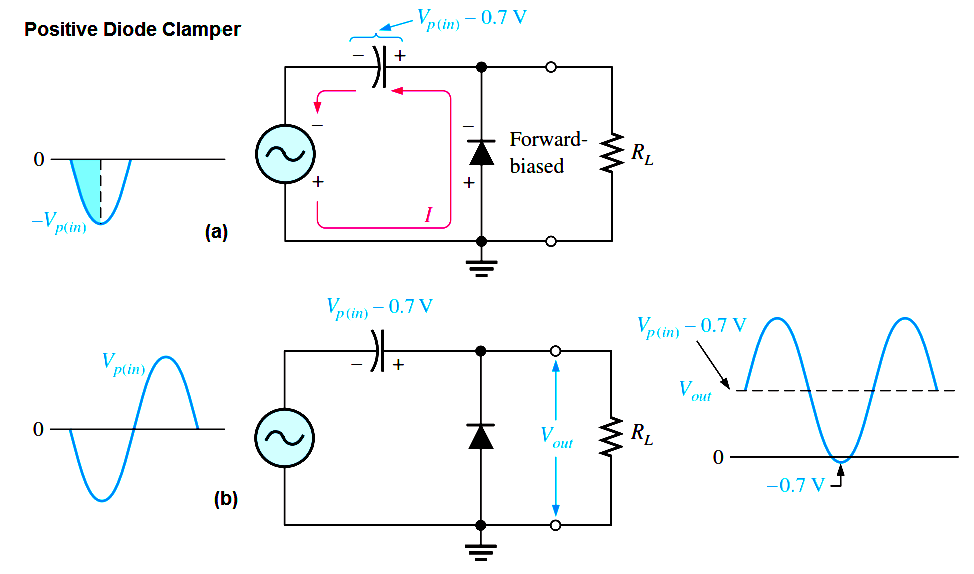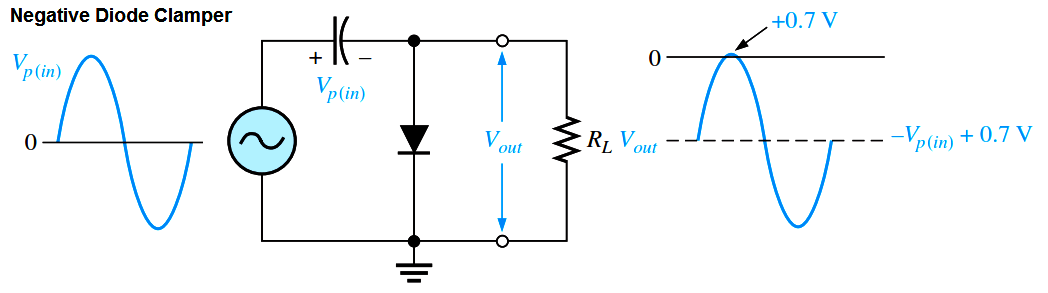# Diode Clampers Principle

A clamper adds a dc level to an ac voltage. Clampers are sometimes known as dc restorers. Below Figure shows a diode clamper that inserts a positive dc level in the output wave form. The operation of this circuit can be seen by considering the first negative half-cycle of the input voltage.

When the input voltage initially goes negative, the diode is forward biased, allowing the capacitor to charge to near the peak of the input (Vp(in) – 0.7 V), as shown in Figure (a). Just after the negative peak, the diode is reverse-biased. This is because the cathode is held near Vp(in) – 0.7 V by the charge on the capacitor. The capacitor can only discharge through the high resistance of RL. So, from the peak of one negative half-cycle to the next, the capacitor discharges very little. The amount that is discharged, of course, depends on the value of RL.Fig : Positive Diode Clamper Operation

If the capacitor discharges during the period of the input wave, clamping action is affected. If the RC time constant is 100 times the period, the clamping action is excellent. An RC time constant of ten times the period will have a small amount of distortion at the ground level due to the charging current.

The net effect of the clamping action is that the capacitor retains a charge approximately equal to the peak value of the input less the diode drop. The capacitor voltage acts essentially as a battery in series with the input voltage. The dc voltage of the capacitor adds to the input voltage by superposition, as in Above Figure. If the diode is turned around, a negative dc voltage is added to the input voltage to produce the output voltage as shown in Below Figure.Fig : Negative Diode Clamper Operation